# Lesson Notes By Weeks and Term - Primary 4

Plane shape

WEEK� 7

SUBJECT: MATHEMATICS

CLASS: PRIMARY 4

TERM: 3RD TERM

TOPIC: Plane shape

SUBTOPIC:�� Symmetrical plane shape.

BEHAVIOURAL OBJECTIVES: At the end of the lesson, pupils should be able to:

1. State the meaning of symmetry
2. Identify Symmetrical plane shape
3. Locate line of symmetry of plane figures at school and home.
4. Identify right angle,acute and obtuse andle in a plane shape.
5. Distinguish between horizontal and vertical.

CONTENT

symmetry means that one shape becomes exactly like another when you move it in some way: turn, flip or slide. For two objects to be symmetrical, they must be the same size and shape, with one object having a different orientation from the first. There can also be symmetry in one object, such as a face.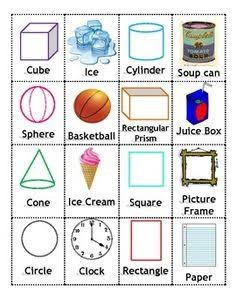SYMMETRICAL PLANE SHAPE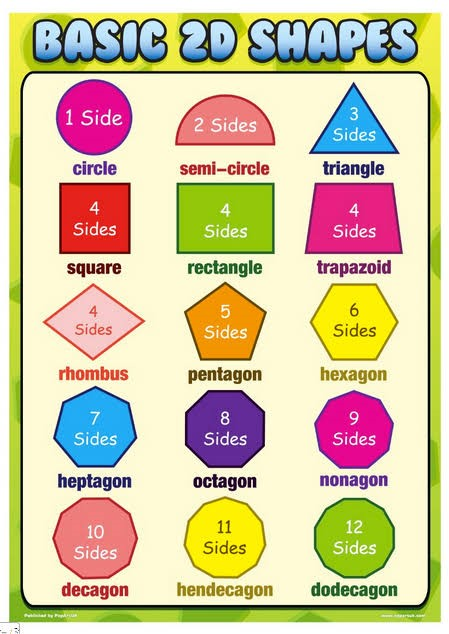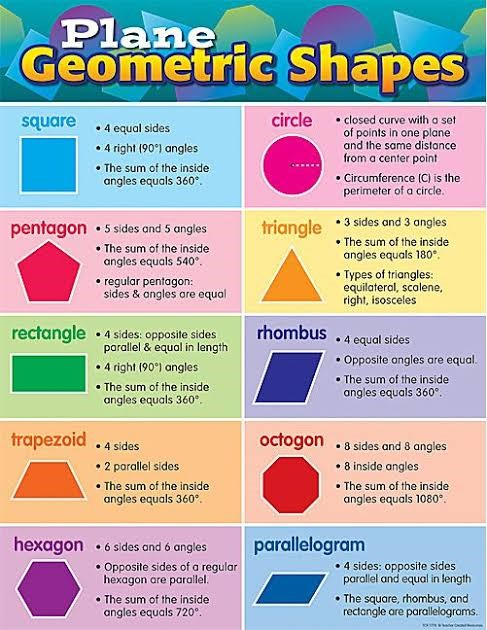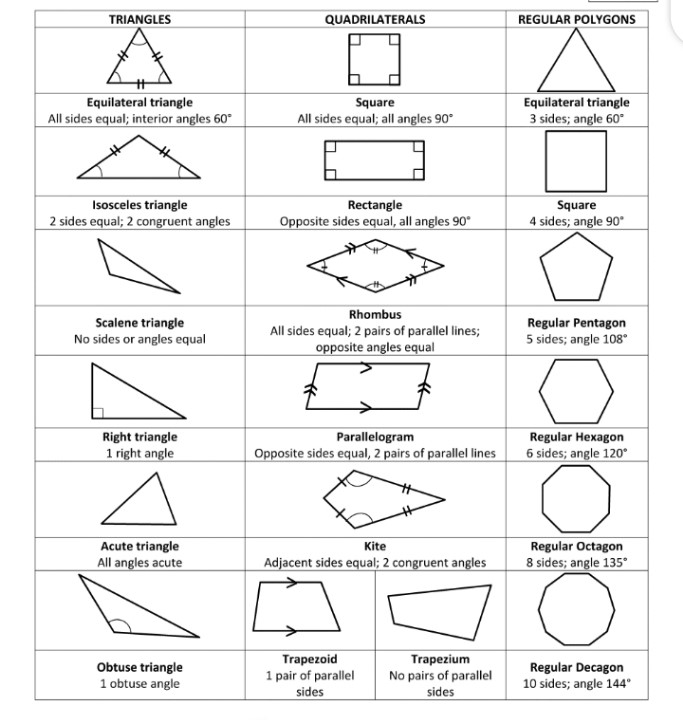Right angle, acute angle and obtuse angle

RIGHT ANGLE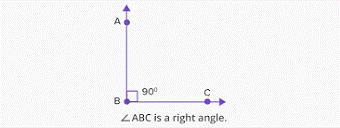Acute angleObtuse angleExercise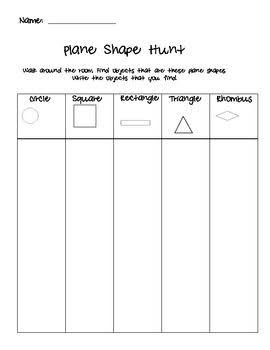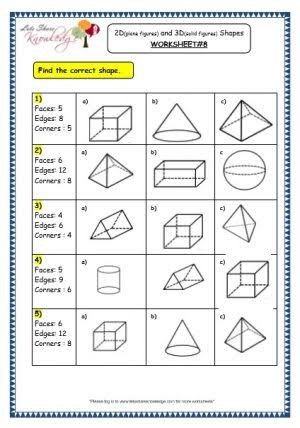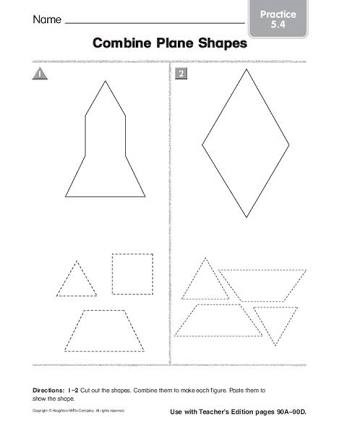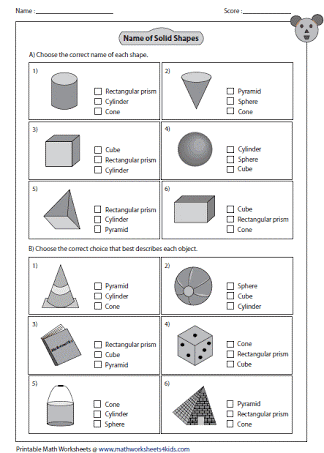The secondary cardinal points

Between North and East, the direction is called North East (NE)

Between South and East, the direction is called South East (SE)

Between South and West, the direction is called South West (SW)

Between North and West, the direction is called North West (NW)

Thus; NE, SE, SW and NW are called the secondary cardinal points.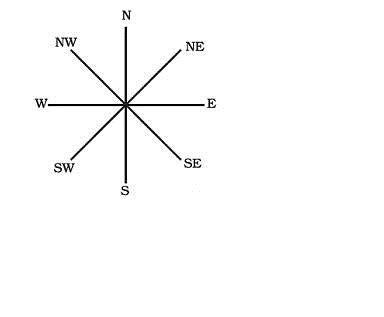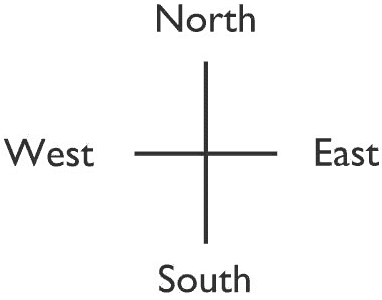Exercise

From the North direction, record the direction the compass pointer turns through in a

clockwise direction:

1. 11/2

right angles = South East (SE) 2. 1/2

right angle = _______________

1. 2 right angles = _______________ 4. 3 right angles = _______________
2. 21/2

right angles = _______________ 6. 31/2

right angles = _______________

1. How many right angles are turned through by facing:
2. North and turn clockwise to face South?
3. West and turn clockwise to face North East?
4. South and turn clockwise to face North East?
5. North and turn anti clockwise to face East?
6. North and turn anti clockwise to face South East?

Copy and complete this table.

Name of shape Number of lines of symmetry

1. a) Trapezium
2. b) Kite
3. c) Parallelogram
4. d) Rhombus
5. e) Equilateral triangle
6. f) Right-angled triangle
7. g) Isosceles triangles
8. h) Circle

Copy and complete.

1. a) A plane shape with all its four sides equal is a
2. b) An equilateral triangle has ____________ equal sides.
3. c) An isosceles triangle has ____________ line of symmetry.
4. d) A rhombus has ____________ lines of symmetry.
5. e) A rectangle has ____________ lines of symmetry.
6. f) A square has ____________ lines of symmetry.
7. g) A kite has ____________ line of symmetry.
8. h) A parallelogram has ____________ line of symmetry.
9. i) A circle has ____________ lines of symmetry.

Draw each of the following shapes and show their line(s) of symmetry.

1. Isosceles trapezium 14. Square 15. Rhombus 16. Rectangle

Unit 3 Properties of parallelogram, rhombus, kite and trapezium

A. Properties of parallelogram

Parallelogram is described as a rectangle pushed over

1. A parallelogram has four sides.
3. Opposite sides are equal.
4. Two opposite sides are parallel.
5. Angles are not right angles.
6. Opposite angles are equal.
7. Diagonals are not equal but bisect each other.
8. No line of symmetry.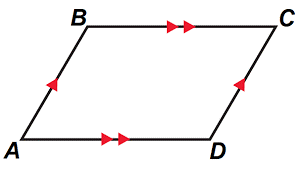B. Properties of a rhombus

A rhombus is described as a square pushed over.

1. A rhombus has four sides.
3. All four sides are equal.
4. Two opposite sides are parallel.
5. Diagonals not equal but bisect each other.
6. Angles not right angles.
7. Opposite angles are equal.
8. Two lines of symmetry.Properties of a kite

1. A kite has four sides.
3. Two sides next to each other are equal.
4. No sides is parallel.
5. It has one line of symmetry.Exercise

1. Write down two properties of
2. a) rhombus b) kite
3. All the properties of the parallelogram and rhombus are the same except in two

properties. Mention the two properties that are different.

1. How would you describe a quadrilateral? Give 2 examples.
2. Draw and name four plane shapes.
3. Using dotted lines show the line or lines of symmetry in each shape.
4. a) When do we say an object is symmetrical?
5. b) Give two examples of symmetrical objects.
6. Draw four letters of the alphabet with their lines of symmetry.
7. List the ones with no lines of symmetry. Only 5
8. How many lines of symmetry does
9. a) an equilateral triangle have?

b) an isosceles triangle have?